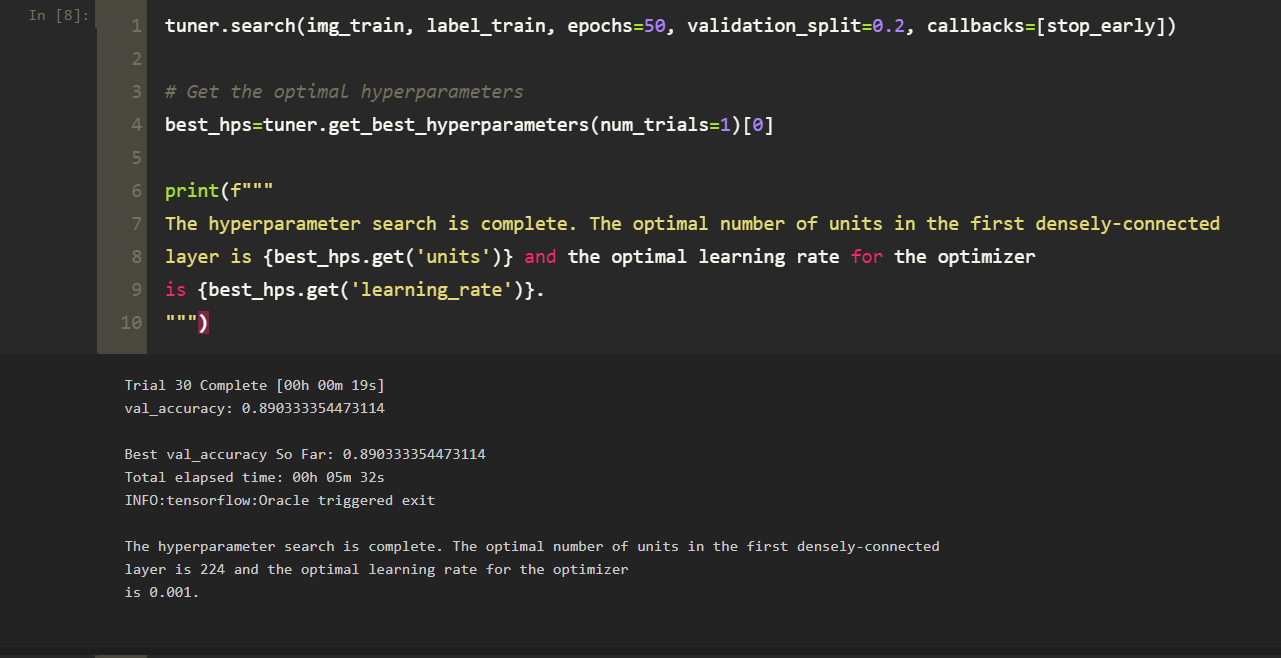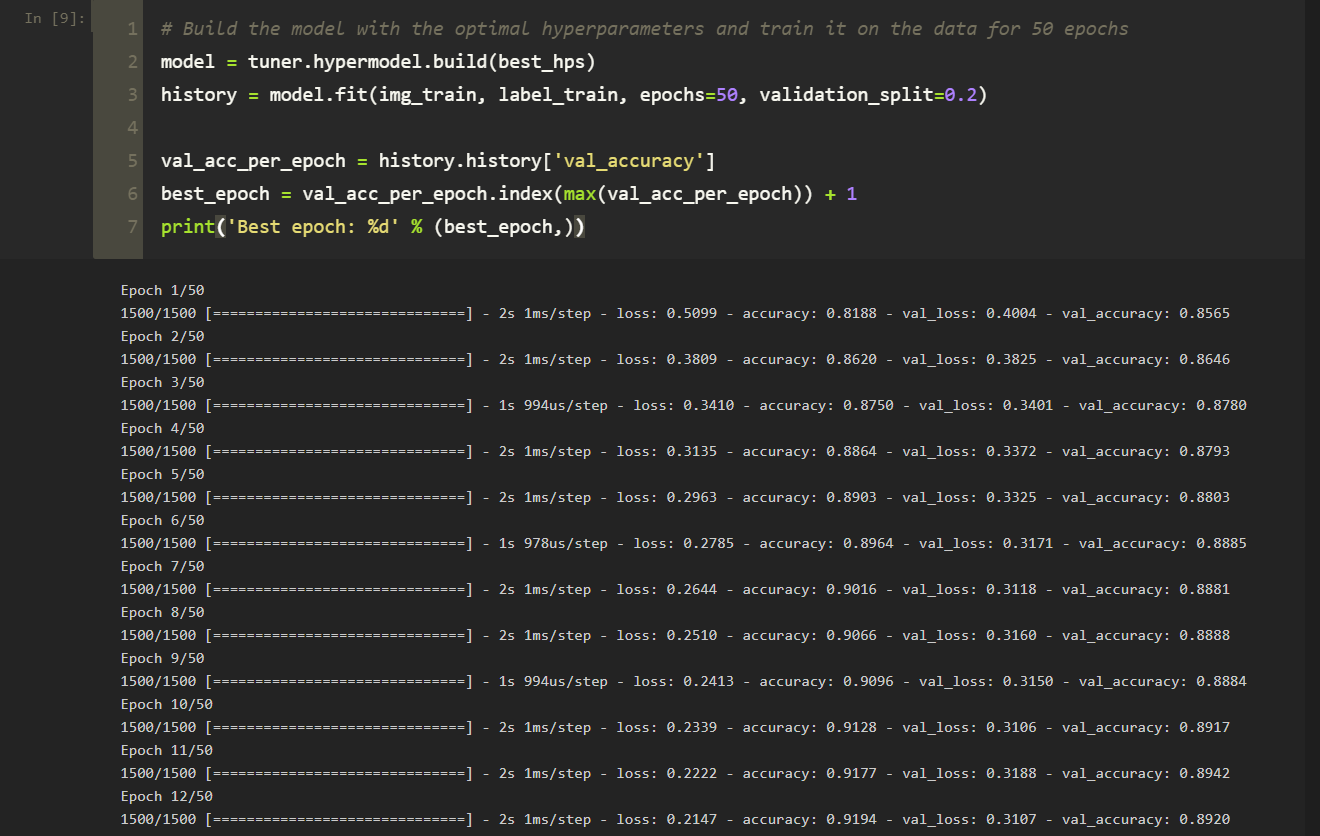# Optimizing Hyperparameters Using The Keras Tuner Framework

Hyperparameter optimization is an integral part of deep learning as a machine learning project is crucially dependent on the choice of good hyperparameters. Neural networks are challenging to configure, and there are a lot of parameters to be set. A good hyperparameter combination can highly improve the model’s performance. Effective hyperparameter search is the missing piece of the puzzle that helps us train our model towards the desired results. As the field of machine learning continues to mature, relying on trial and error to find good hyperparameter values isn’t a scalable approach. Many of today’s state-of-the-art models were discovered via sophisticated hyperparameter optimization algorithms.

What are Hyperparameters?

Hyperparameters are the variables that overlook the training process of an ML model. These variables remain constant over the training process impacting the performance of the model. Alternatively, Hyperparameters are the knobs that you can turn when building your model. Hyperparameters are of two types:

1. Model hyperparameters influence model selection, such as the number and width of hidden layers.
2. Algorithm hyperparameters that influence the learning algorithm’s speed and quality, such as Gradient Descent’s learning rate and the number of nearest neighbors for a k Nearest Neighbors (KNN) classifier.

What is Keras Tuner?

Keras Tuner is an easy-to-use hyperparameter optimization framework that solves the pain points of performing a hyperparameter search. It helps to find optimal hyperparameters for an ML model. Keras Tuner makes it easy to define a search space and work with algorithms to find the best hyperparameter values. Keras Tuner comes with built-in Bayesian Optimization, Hyperband, and Random Search algorithms and is easily extendable to experiment with other algorithms.

Random Search Algorithm

The algorithm sets up a grid of hyperparameter values and selects random combinations to train the model where The number of search iterations is set based on time and resources. Random search is much more efficient than grid search, where all the possible combinations from the grid are tried until the best combination is found. Although grid search finds the optimal values of hyperparameters, the random search usually considers a good enough combination in far fewer iterations.

Hyperband Algorithm

Hyperband is an optimized variation of random search which uses early-stopping to speed up the process. The underlying principle of the procedure exploits the idea that if a hyperparameter configuration is expected to be the best after a considerable number of iterations, it is more likely to perform after a small number of iterations. The main idea is to fit many models for a small number of epochs and only to continue training for the models achieving the highest accuracy on the validation set.

Bayesian Optimisation Algorithm

Bayesian optimization aims to become less wrong with more data inputs done by continually updating the surrogate probability model after each objective function’s evaluation epoch. The algorithm Builds a surrogate probability model of the objective function and finds the hyperparameters that perform best on the surrogate. The hyperparameters acquired are applied to the actual objective function, and the algorithm is run till the desired results are not achieved.

Implementation of Hypertuning

Write the following code to perform hyper tuning on an image classification model using Keras Tuner:

``````import tensorflow as tf
from tensorflow import keras
import kerastuner as kt

(img_train, label_train), (img_test, label_test) = keras.datasets.fashion_mnist.load_data()
# Normalize pixel values between 0 and 1
img_train = img_train.astype('float32') / 255.0
img_test = img_test.astype('float32') / 255.0

def model_builder(hp):
model = keras.Sequential()

# Tune the number of units in the first Dense layer
# Choose an optimal value between 32-512
hp_units = hp.Int('units', min_value=32, max_value=512, step=32)

# Tune the learning rate for the optimizer
# Choose an optimal value from 0.01, 0.001, or 0.0001
hp_learning_rate = hp.Choice('learning_rate', values=[1e-2, 1e-3, 1e-4])

loss=keras.losses.SparseCategoricalCrossentropy(from_logits=True),
metrics=['accuracy'])

return model

tuner = kt.Hyperband(model_builder,
objective='val_accuracy',
max_epochs=10,
factor=3,
directory='my_dir',
project_name='intro_to_kt')

stop_early = tf.keras.callbacks.EarlyStopping(monitor='val_loss', patience=5)

tuner.search(img_train, label_train, epochs=50, validation_split=0.2, callbacks=[stop_early])

# Get the optimal hyperparameters
best_hps=tuner.get_best_hyperparameters(num_trials=1)

print(f"""
The hyperparameter search is complete. The optimal number of units in the first densely-connected
layer is {best_hps.get('units')} and the optimal learning rate for the optimizer
is {best_hps.get('learning_rate')}.
""")

# Build the model with the optimal hyperparameters and train it on the data for 50 epochs
model = tuner.hypermodel.build(best_hps)
history = model.fit(img_train, label_train, epochs=50, validation_split=0.2)

val_acc_per_epoch = history.history['val_accuracy']
best_epoch = val_acc_per_epoch.index(max(val_acc_per_epoch)) + 1
print('Best epoch: %d' % (best_epoch,))

hypermodel = tuner.hypermodel.build(best_hps)

# Retrain the model with the best epoch
hypermodel.fit(img_train, label_train, epochs=best_epoch, validation_split=0.2)

eval_result = hypermodel.evaluate(img_test, label_test)
print("[test loss, test accuracy]:", eval_result)``````